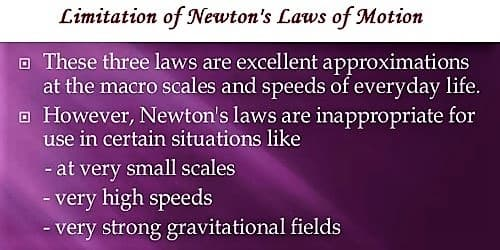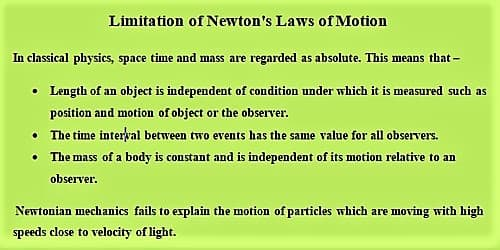# Limitation of Newton’s Laws of Motion

Limitation of Newton’s Laws of Motion

As a matter of fact, Newton’s first law is related to a frame of reference. Because generally, the acceleration of a body measured with reference to a frame of reference depends on that reference. The first law states that, if there is nobody available nearby, then a family of frame of reference could be obtained whereat no acceleration of a particle exists. Newton’s first law says that if there is acceleration, there is a force, but there are no forces acting on the object because we know it is static. Major two limitations are, Force required when an object changes motion and Acceleration is the observable consequence of forces acting. In the absence of applied force bodies remain in the state of rest or maintain uniform linear motion- this property is nothing but inertia.

From Newton’s second law of motion, we have learned that the acceleration of a given body is proportional to the applied force on it. Now our question is whether the same force on other bodies will be different or not. The remaining significant question is: will the same force acting on different bodies be different? That means what kind of action is applied by the same force on different bodies. For his Second Law to be valid at high speeds it needs to be written in its original form rather than the present general form of ‘F = ma’. Newton’s second law is not appropriate in the case of non-inertial frames, and the third law is violated in relativity.

As far as we know, there are no limitations to Newton’s third law. Each and every force comes with equivalent and conflicting force acting anywhere in the universe. His Third Law is often said to be corresponding to Conservation of Momentum and for this to be true in Modern Physics we need to understand that various ‘fields’ have momentum as well as do particles.

From this law, we get a qualitative answer to it. From Newton’s third law we learn that a unified force is simply the direction of the interaction between two bodies. Further, we see that the magnitudes of these two forces are equal but oppositely directed. So, there is no existence of an insulated or isolated force- it is impossible to get it.Physics is not of a combination of rigid theories — rather it is a continuously developing science. It is seen that physics started attaining perfection in 1660 by Newtonian mechanics, in 1870 by Maxwell’s electromagnetic theory, in 1905 by Einstein’s theory of relativity and in 1925 by quantum mechanics. It is seen that theories of Newtonian mechanics are applicable in a limited area and has some limitations.

During the last few decades, it has been possible to measure properties of tiny speeds particles like electron, proton and other fundamental particles by quantum mechanics. Newtonian mechanics could not describe the motion of such speedy particles. Newtonian mechanics is very suitable in explaining difficult phenomena at, v/c « 1; but collision, decay, and interaction of fundamental particles having high speed cannot be explained by Newtonian mechanics.

Even then the importance of Newtonian mechanics is not least. Newtonian mechanics may be considered as a special case of the general mechanics that deals with particles having the speed of that of light. The mass of those materials which we deal with in our daily life is much higher than that of an electron (mass of electron, m = 9.1 x 10-31 kg). It is a fascinating matter that the concept of extremely close relation with ‘particle’ is the foundation of classical mechanics.

By this mechanics or by Newton’s equations of motion it is not possible to measure position ‘x’ of particles and their velocity ‘v’ simultaneously and accurately. There is uncertainty. This uncertainty is known as Heisenberg’s uncertainty principle which is expressed as

∆x ≈ h/(m∆vx); here, h = Plank’s constant.

Newtonian mechanics is a special form of a general theory which fails to explain the behavior of small particles which have been done by quantum mechanics developed by, Heisenberg, Schrodinger, Barn in 1925-1926 and Dirac in 1927 and other physicists.